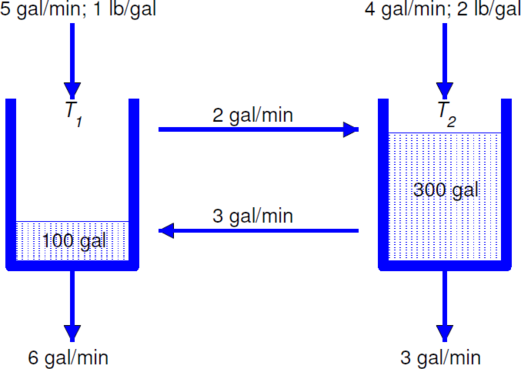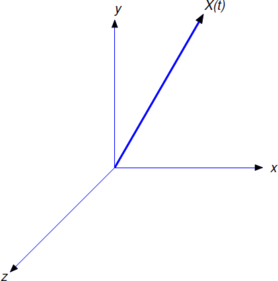$$\newcommand{\id}{\mathrm{id}}$$ $$\newcommand{\Span}{\mathrm{span}}$$ $$\newcommand{\kernel}{\mathrm{null}\,}$$ $$\newcommand{\range}{\mathrm{range}\,}$$ $$\newcommand{\RealPart}{\mathrm{Re}}$$ $$\newcommand{\ImaginaryPart}{\mathrm{Im}}$$ $$\newcommand{\Argument}{\mathrm{Arg}}$$ $$\newcommand{\norm}{\| #1 \|}$$ $$\newcommand{\inner}{\langle #1, #2 \rangle}$$ $$\newcommand{\Span}{\mathrm{span}}$$

# 10.1: Introduction to Systems of Differential Equations

•• Contributed by William F. Trench
• Andrew G. Cowles Distinguished Professor Emeritus (Mathamatics) at Trinity University
$$\newcommand{\vecs}{\overset { \rightharpoonup} {\mathbf{#1}} }$$ $$\newcommand{\vecd}{\overset{-\!-\!\rightharpoonup}{\vphantom{a}\smash {#1}}}$$$$\newcommand{\id}{\mathrm{id}}$$ $$\newcommand{\Span}{\mathrm{span}}$$ $$\newcommand{\kernel}{\mathrm{null}\,}$$ $$\newcommand{\range}{\mathrm{range}\,}$$ $$\newcommand{\RealPart}{\mathrm{Re}}$$ $$\newcommand{\ImaginaryPart}{\mathrm{Im}}$$ $$\newcommand{\Argument}{\mathrm{Arg}}$$ $$\newcommand{\norm}{\| #1 \|}$$ $$\newcommand{\inner}{\langle #1, #2 \rangle}$$ $$\newcommand{\Span}{\mathrm{span}}$$ $$\newcommand{\id}{\mathrm{id}}$$ $$\newcommand{\Span}{\mathrm{span}}$$ $$\newcommand{\kernel}{\mathrm{null}\,}$$ $$\newcommand{\range}{\mathrm{range}\,}$$ $$\newcommand{\RealPart}{\mathrm{Re}}$$ $$\newcommand{\ImaginaryPart}{\mathrm{Im}}$$ $$\newcommand{\Argument}{\mathrm{Arg}}$$ $$\newcommand{\norm}{\| #1 \|}$$ $$\newcommand{\inner}{\langle #1, #2 \rangle}$$ $$\newcommand{\Span}{\mathrm{span}}$$

$$\newcommand{\place}{\bigskip\hrule\bigskip\noindent} \newcommand{\threecol}{\left[\begin{array}{r}#1\\#2\\#3\end{array}\right]} \newcommand{\threecolj}{\left[\begin{array}{r}#1\1\jot]#2\\[1\jot]#3\end{array}\right]} \newcommand{\lims}{\,\bigg|_{#1}^{#2}} \newcommand{\twocol}{\left[\begin{array}{l}#1\\#2\end{array}\right]} \newcommand{\ctwocol}{\left[\begin{array}{c}#1\\#2\end{array}\right]} \newcommand{\cthreecol}{\left[\begin{array}{c}#1\\#2\\#3\end{array}\right]} \newcommand{\eqline}{\centerline{\hfill\displaystyle#1\hfill}} \newcommand{\twochar}{\left|\begin{array}{cc} #1-\lambda\\#3-\lambda\end{array}\right|} \newcommand{\twobytwo}{\left[\begin{array}{rr} #1\\#3\end{array}\right]} \newcommand{\threechar}{\left[\begin{array}{ccc} #1-\lambda\\#4-\lambda\\#7 -\lambda\end{array}\right]} \newcommand{\threebythree}{\left[\begin{array}{rrr} #1\\#4\\#7 \end{array}\right]} \newcommand{\solutionpart}{\vskip10pt\noindent\underbar{\color{blue}\sc Solution({\bf #1})\ }} \newcommand{\Cex}{\fbox{\textcolor{red}{C}}\, } \newcommand{\CGex}{\fbox{\textcolor{red}{C/G}}\, } \newcommand{\Lex}{\fbox{\textcolor{red}{L}}\, } \newcommand{\matfunc}{\left[\begin{array}{cccc}#1_{11}(t)_{12}(t)&\cdots _{1#3}(t)\\#1_{21}(t)_{22}(t)&\cdots_{2#3}(t)\\\vdots& \vdots&\ddots&\vdots\\#1_{#21}(t)_{#22}(t)&\cdots_{#2#3}(t) \end{array}\right]} \newcommand{\col}{\left[\begin{array}{c}#1_1\\#1_2\\\vdots\\#1_#2\end{array}\right]} \newcommand{\colfunc}{\left[\begin{array}{c}#1_1(t)\\#1_2(t)\\\vdots\\#1_#2(t)\end{array}\right]} \newcommand{\cthreebythree}{\left[\begin{array}{ccc} #1\\#4\\#7 \end{array}\right]} 1 \ newcommand {\ dy} {\ ,\ mathrm {d}y} \ newcommand {\ dx} {\ ,\ mathrm {d}x} \ newcommand {\ dyx} {\ ,\ frac {\ mathrm {d}y}{\ mathrm {d}x}} \ newcommand {\ ds} {\ ,\ mathrm {d}s} \ newcommand {\ dt }{\ ,\ mathrm {d}t} \ newcommand {\dst} {\ ,\ frac {\ mathrm {d}s}{\ mathrm {d}t}}$$ Many physical situations are modelled by systems of $$n$$ differential equations in $$n$$ unknown functions, where $$n\ge2$$. The next three examples illustrate physical problems that lead to systems of differential equations. In these examples and throughout this chapter we’ll denote the independent variable by $$t$$. Example $$\PageIndex{1}$$: Flow Tanks $$T_1$$ and $$T_2$$ contain 100 gallons and 300 gallons of salt solutions, respectively. Salt solutions are simultaneously added to both tanks from external sources, pumped from each tank to the other, and drained from both tanks (Figure $$\PageIndex{1}$$ ). A solution with $$1$$ pound of salt per gallon is pumped into $$T_1$$ from an external source at $$5$$ gal/min, and a solution with $$2$$ pounds of salt per gallon is pumped into $$T_2$$ from an external source at $$4$$ gal/min. The solution from $$T_1$$ is pumped into $$T_2$$ at 2 gal/min, and the solution from $$T_2$$ is pumped into $$T_1$$ at $$3$$ gal/min. $$T_1$$ is drained at $$6$$ gal/min and $$T_2$$ is drained at 3 gal/min. Let $$Q_1(t)$$ and $$Q_2(t)$$ be the number of pounds of salt in $$T_1$$ and $$T_2$$, respectively, at time $$t>0$$. Derive a system of differential equations for $$Q_1$$ and $$Q_2$$. Assume that both mixtures are well stirred.Figure $$\PageIndex{1}$$ As in Section 4.2, let rate in and rate out denote the rates (lb/min) at which salt enters and leaves a tank; thus, \[\begin{aligned} Q_1'&= (\mbox{rate in})_1-(\mbox{rate out})_1,\\[4pt] Q_2'&= (\mbox{rate in})_2-(\mbox{rate out})_2.\end{aligned}

Note that the volumes of the solutions in $$T_1$$ and $$T_2$$ remain constant at 100 gallons and 300 gallons, respectively.

$$T_1$$ receives salt from the external source at the rate of

$\mbox{(1 lb/gal) }\times\mbox{ (5~gal/min)}=\mbox{ 5 lb/min}, \nonumber$

and from $$T_2$$ at the rate of

$\mbox{(lb/gal in }T_2)\times\mbox{ (3~gal/min) }={1\over300}Q_2\times3\\[4pt]={1\over100}Q_2 \mbox{ lb/min}.\nonumber$

Therefore

$\label{eq:10.1.1} \mbox{(rate in)}_1= 5+{1\over100}Q_2.$

Solution leaves $$T_1$$ at the rate of 8 gal/min, since 6 gal/min are drained and 2 gal/min are pumped to $$T_2$$; hence,

$\label{eq:10.1.2} (\mbox{rate out})_1=(\mbox{ lb/gal in T}_1)\times \mbox{(8~gal/min) } ={1\over100}Q_1\times8={2\over25}Q_1.$

Equations \ref{eq:10.1.1} and Equation \ref{eq:10.1.2} imply that

$\label{eq:10.1.3} Q_1'=5+{1\over100}Q_2-{2\over25}Q_1.$

$$T_2$$ receives salt from the external source at the rate of

$\mbox{(2 lb/gal) }\times\mbox{ (4~gal/min)}=\mbox{ 8 lb/min},\nonumber$

and from $$T_1$$ at the rate of

$\mbox{(lb/gal in }T_1)\times\mbox{ (2~gal/min) }={1\over100}Q_1\times2\\[4pt]={1\over50}Q_1 \mbox{ lb/min}.\nonumber$

Therefore

$\label{eq:10.1.4} \mbox{(rate in)}_2= 8+{1\over50}Q_1.$

Solution leaves $$T_2$$ at the rate of $$6$$ gal/min, since $$3$$ gal/min are drained and $$3$$ gal/min are pumped to $$T_1$$; hence,

$\label{eq:10.1.5} (\mbox{rate out})_2=(\mbox{ lb/gal in T}_2)\times \mbox{(6~gal/min) } ={1\over300}Q_2\times6={1\over50}Q_2.$

Equations \ref{eq:10.1.4} and \ref{eq:10.1.5} imply that

$\label{eq:10.1.6} Q_2'=8+{1\over50}Q_1-{1\over50}Q_2.$

We say that Equations \ref{eq:10.1.3} and \ref{eq:10.1.6} form a system of two first order equations in two unknowns, and write them together as

\begin{align*} Q_1'&= 5- \dfrac{2}{25}Q_1 + \dfrac{1}{100} Q_2 \\ Q_2' &= 8+ \dfrac{1}{50} Q_1 - \dfrac{1}{50} Q_2. \end{align*}

Example $$\PageIndex{2}$$: Coupled Springs

A mass $$m_1$$ is suspended from a rigid support on a spring $$S_1$$ and a second mass $$m_2$$ is suspended from the first on a spring $$S_2$$ (Figure $$\PageIndex{2}$$). The springs obey Hooke’s law, with spring constants $$k_1$$ and $$k_2$$. Internal friction causes the springs to exert damping forces proportional to the rates of change of their lengths, with damping constants $$c_1$$ and $$c_2$$. Let $$y_1=y_1(t)$$ and $$y_2=y_2(t)$$ be the displacements of the two masses from their equilibrium positions at time $$t$$, measured positive upward. Derive a system of differential equations for $$y_1$$ and $$y_2$$, assuming that the masses of the springs are negligible and that vertical external forces $$F_1$$ and $$F_2$$ also act on the objects.

Solution

In equilibrium, $$S_1$$ supports both $$m_1$$ and $$m_2$$ and $$S_2$$ supports only $$m_2$$. Therefore, if $$\Delta\ell_1$$ and $$\Delta\ell_2$$ are the elongations of the springs in equilibrium then

$\label{eq:10.1.7} (m_1+m_2)g=k_1\Delta\ell_1\quad\mbox{ and }\quad m_2g=k_2\Delta\ell_2.$Figure $$\PageIndex{2}$$

Let $$H_1$$ be the Hooke’s law force acting on $$m_1$$, and let $$D_1$$ be the damping force on $$m_1$$. Similarly, let $$H_2$$ and $$D_2$$ be the Hooke’s law and damping forces acting on $$m_2$$. According to Newton’s second law of motion,

$\label{eq:10.1.8} \begin{array}{ccl} m_1y_1''=-m_1g+H_1+D_1+F_1,\\[4pt] m_2y_2''=-m_2g+H_2+D_2+F_2. \end{array}$

When the displacements are $$y_1$$ and $$y_2$$, the change in length of $$S_1$$ is $$-y_1+\Delta\ell_1$$ and the change in length of $$S_2$$ is $$-y_2+y_1+\Delta\ell_2$$. Both springs exert Hooke’s law forces on $$m_1$$, while only $$S_2$$ exerts a Hooke’s law force on $$m_2$$. These forces are in directions that tend to restore the springs to their natural lengths. Therefore

$\label{eq:10.1.9} H_1=k_1(-y_1+\Delta\ell_1)-k_2(-y_2+y_1+\Delta\ell_2)\quad\mbox{ and }\quad H_2=k_2(-y_2+y_1+\Delta\ell_2).$

When the velocities are $$y_1'$$ and $$y_2'$$, $$S_1$$ and $$S_2$$ are changing length at the rates $$-y_1'$$ and $$-y_2'+y_1'$$, respectively. Both springs exert damping forces on $$m_1$$, while only $$S_2$$ exerts a damping force on $$m_2$$. Since the force due to damping exerted by a spring is proportional to the rate of change of length of the spring and in a direction that opposes the change, it follows that

$\label{eq:10.1.10} D_1=-c_1y_1'+c_2(y_2'-y_1')\quad\mbox{ and }\quad D_2=-c_2(y_2'-y_1').$

From Equations \ref{eq:10.1.8}, \ref{eq:10.1.9}, and \ref{eq:10.1.10},

$\label{eq:10.1.11} \begin{array} {m_1y_1''}&{= -m_1g+k_1(-y_1+\Delta\ell_1)-k_2(-y_2+y_1+\Delta\ell_2) -c_1y_1'+c_2(y_2'-y_1')+F_1}\\ {}&{= -(m_1g-k_1\Delta\ell_1+k_2\Delta\ell_2)-k_1y_1+k_2(y_2-y_1) -c_1y_1'+c_2(y_2'-y_1')+F_1} \end{array}$

and

$\label{eq:10.1.12} \begin{array}{ccl} m_2y_2''&= -m_2g+k_2(-y_2+y_1+\Delta\ell_2)-c_2(y_2'-y_1')+F_2\\[4pt] &= -(m_2g-k_2\Delta\ell_2)-k_2(y_2-y_1)-c_2(y_2'-y_1')+F_2. \end{array}$

From Equation \ref{eq:10.1.7},

$m_1g-k_1\Delta\ell_1+k_2\Delta\ell_2=-m_2g+k_2\Delta\ell_2=0. \nonumber$

Therefore we can rewrite Equation \ref{eq:10.1.11} and Equation \ref{eq:10.1.12} as

\begin{align*} m_1y_1''&= -(c_1+c_2)y_1'+c_2y_2'-(k_1+k_2)y_1+k_2y_2+F_1\\ m_2y_2''&= c_2y_1'-c_2y_2'+k_2y_1-k_2y_2+F_2. \quad \end{align*}

Example $$\PageIndex{3}$$: Gravity

Let $${\bf X}={\bf X}(t)=x(t)\,{\bf i}+y(t)\,{\bf j}+z(t)\,{\bf k}$$ be the position vector at time $$t$$ of an object with mass $$m$$, relative to a rectangular coordinate system with origin at Earth’s center (Figure $$\PageIndex{3}$$).Figure $$\PageIndex{3}$$

According to Newton’s law of gravitation, Earth’s gravitational force $${\bf F}={\bf F}(x,y,z)$$ on the object is inversely proportional to the square of the distance of the object from Earth’s center, and directed toward the center; thus,

$\label{eq:10.1.13} \bf {F}= \dfrac{K}{ || \bf {X} ||^2} \left(- \dfrac{ \bf {X}} {|| \bf {X} ||} \right) = -K {x\,{\bf i}+y\,{\bf j}+z\,{\bf k}\over\left(x^2+y^2+z^2\right)^{3/2}},$

where $$K$$ is a constant. To determine $$K$$, we observe that the magnitude of $${\bf F}$$ is

$\|{\bf F}\|=K{\|{\bf X}\|\over\|{\bf X}\|^3}={K\over\|{\bf X}\|^2} ={K\over(x^2+y^2+z^2)}. \nonumber$

Let $$R$$ be Earth’s radius. Since $$\|{\bf F}\|=mg$$ when the object is at Earth’s surface,

$mg = {K\over R^2},\quad\mbox{ so }\quad K=mgR^2. \nonumber$

Therefore we can rewrite Equation \ref{eq:10.1.13} as

${\bf F}=-mgR^2{x\,{\bf i}+y\,{\bf j}+z\,{\bf k}\over\mbox{}\left(x^2+y^2+z^2\right)^{3/2}}. \nonumber$

Now suppose $${\bf F}$$ is the only force acting on the object. According to Newton’s second law of motion, $${\bf F}=m{\bf X}''$$; that is,

$m(x''\,{\bf i}+y''\,{\bf j}+z''\,{\bf k})= -mgR^2{x\,{\bf i}+y\,{\bf j}+z\,{\bf k}\over\left(x^2+y^2+z^2\right)^{3/2}}. \nonumber$

Cancelling the common factor $$m$$ and equating components on the two sides of this equation yields the system$\label{eq:10.1.14} \begin{array}{ll} {x''}&{= - \dfrac{ gR^2x}{(x^2+y^2+z^2)^{3/2}}} \\ {y''} &{= - \dfrac{gR^2y}{(x^2+y^2+z^2)^{3/2}}} \\ {z''}&{= - \dfrac{gR^2z}{(x^2+y^2+z^2)^{3/2}}.} \end{array}$

## Rewriting Higher Order Systems as First Order Systems

A system of the form

$\label{eq:10.1.15} \begin{array}{ccl} y_1'&= g_1(t,y_1,y_2,\dots,y_n)\\ y_2'&= g_2(t,y_1,y_2,\dots,y_n)\\ &\vdots&\\ y_n'&= g_n(t,y_1,y_2,\dots,y_n) \end{array}$

is called a first order system, since the only derivatives occurring in it are first derivatives. The derivative of each of the unknowns may depend upon the independent variable and all the unknowns, but not on the derivatives of other unknowns. When we wish to emphasize the number of unknown functions in Equation \ref{eq:10.1.15} we will say that Equation \ref{eq:10.1.15} is an $$n\times n$$ system.

Systems involving higher order derivatives can often be reformulated as first order systems by introducing additional unknowns. The next two examples illustrate this.

Example $$\PageIndex{4}$$

Rewrite the system

$\label{eq:10.1.16} \begin{array}{ll} {m_1y_1''}&{= -(c_1+c_2)y_1'+c_2y_2'-(k_1+k_2)y_1+k_2y_2+F_1}\\ {m_2y_2''}&{= c_2y_1'-c_2y_2'+k_2y_1-k_2y_2+F_2.} \end{array}$

derived in Example $$\PageIndex{2}$$ as a system of first order equations.

Solution

If we define $$v_1=y_1'$$ and $$v_2=y_2'$$, then $$v_1'=y_1''$$ and $$v_2'=y_2''$$, so Equation \ref{eq:10.1.16} becomes

\begin{aligned} m_1v_1'&= -(c_1+c_2)v_1+c_2v_2-(k_1+k_2)y_1+k_2y_2+F_1\\[4pt] m_2v_2'&= c_2v_1-c_2v_2+k_2y_1-k_2y_2+F_2. \end{aligned}\nonumber

Therefore $$\{y_1,y_2,v_1,v_2\}$$ satisfies the $$4\times4$$ first order system

$\label{eq:10.1.17} \begin{array}{ll} {y_1'} &{= v_1} \\ {y_2'} &{= v_2} \\ {v_1'} &{= \dfrac{1}{m_1} \left[-(c_1+c_2)v_1+c_2v_2-(k_1+k_2)y_1+k_2y_2+F_1\right]} \\ {v_2'}&{= \dfrac{1}{m_2} \left[c_2v_1-c_2v_2+k_2y_1-k_2y_2+F_2\right].} \end{array}$

Note

The difference in form between Equation \ref{eq:10.1.15} and Equation \ref{eq:10.1.17}, due to the way in which the unknowns are denoted in the two systems, isn’t important; Equation \ref{eq:10.1.17} is a first order system, in that each equation in Equation \ref{eq:10.1.17} expresses the first derivative of one of the unknown functions in a way that does not involve derivatives of any of the other unknowns.

Example $$\PageIndex{5}$$

Rewrite the system

$\begin{array}{ccc} x''&= f(t,x,x',y,y',y'')\\[4pt]y'''&= g(t,x,x',y,y'y'') \end{array}\nonumber$

as a first order system.

Solution

We regard $$x$$, $$x'$$, $$y$$, $$y'$$, and $$y''$$ as unknown functions, and rename them

$x=x_1,\; x'=x_2,\quad y=y_1,\quad y'=y_2,\quad y''=y_3.\nonumber$

These unknowns satisfy the system

\begin{aligned} x_1'&= x_2\\[4pt]x_2' &= f(t,x_1,x_2,y_1,y_2,y_3) \\[4pt] y_1' &= y_2\\[4pt] y_2' &= y_3 \\[4pt] y_3'&= g(t,x_1,x_2,y_1,y_2,y_3).\end{aligned}\nonumber

## Rewriting Scalar Differential Equations as Systems

In this chapter we’ll refer to differential equations involving only one unknown function as scalar differential equations. Scalar differential equations can be rewritten as systems of first order equations by the method illustrated in the next two examples.

Example $$\PageIndex{6}$$

Rewrite the equation

$\label{eq:10.1.18} y^{(4)}+4y'''+6y''+4y'+y=0$

as a $$4\times4$$ first order system.

Solution

We regard $$y$$, $$y'$$, $$y''$$, and $$y'''$$ as unknowns and rename them

$y=y_1,\quad y'=y_2,\quad y''=y_3,\quad \text{and} \quad y'''=y_4. \nonumber$

Then $$y^{(4)}=y_4'$$, so Equation \ref{eq:10.1.18} can be written as

$y_4'+4y_4+6y_3+4y_2+y_1=0. \nonumber$

Therefore $$\{y_1,y_2,y_3,y_4\}$$ satisfies the system

\begin{align*} y_1' &= y_2\\ y_2' &= y_3 \\ y_3' &= y_4\\ y_4' &= -4y_4-6y_3-4y_2-y_1. \end{align*}

Example $$\PageIndex{7}$$

Rewrite

$x'''=f(t,x,x',x'') \nonumber$

as a system of first order equations.

Solution

We regard $$x$$, $$x'$$, and $$x''$$ as unknowns and rename them

$x=y_1, \quad x'=y_2,\quad \text{and} \quad x''=y_3. \nonumber$

Then

$y_1'=x'=y_2,\quad y_2'=x''=y_3,\quad \text{and} \quad y_3'=x'''. \nonumber$

Therefore $$\{y_1,y_2,y_3\}$$ satisfies the first order system

\begin{align*} y_1'&= y_2 \\[4pt] y_2' &= y_3\\[4pt] y_3' &= f(t,y_1,y_2,y_3). \end{align*}

Since systems of differential equations involving higher derivatives can be rewritten as first order systems by the method used in Examples $$\PageIndex{5}$$-$$\PageIndex{7}$$ , we’ll consider only first order systems.

## Numerical Solution of Systems

The numerical methods that we studied in Chapter 3 can be extended to systems, and most differential equation software packages include programs to solve systems of equations. We will not go into detail on numerical methods for systems; however, for illustrative purposes we’ll describe the Runge-Kutta method for the numerical solution of the initial value problem

\begin{aligned} y_1'&= g_1(t,y_1,y_2),\quad y_1(t_0)=y_{10},\\[4pt] y_2'&= g_2(t,y_1,y_2),\quad y_2(t_0)=y_{20}\end{aligned}

at equally spaced points $$t_0$$, $$t_1$$, …, $$t_n=b$$ in an interval $$[t_0,b]$$. Thus,

$t_i=t_0+ih,\quad i=0,1,\dots,n, \nonumber$

where

$h={b-t_0\over n}. \nonumber$

We’ll denote the approximate values of $$y_1$$ and $$y_2$$ at these points by $$y_{10},y_{11},\dots,y_{1n}$$ and $$y_{20},y_{21},\dots,y_{2n}$$. The Runge-Kutta method computes these approximate values as follows: given $$y_{1i}$$ and $$y_{2i}$$, compute

\begin{aligned} I_{1i}&= g_1(t_i,y_{1i},y_{2i}),\\[4pt] J_{1i}&= g_2(t_i,y_{1i},y_{2i}),\\[4pt] I_{2i}&= g_1\left(t_i+{h\over2},y_{1i}+{h\over2}I_{1i},y_{2i}+{h\over2}J_{1i}\right),\\[4pt] J_{2i}&= g_2\left(t_i+{h\over2},y_{1i}+{h\over2}I_{1i},y_{2i}+{h\over2}J_{1i}\right),\\[4pt] I_{3i}&= g_1\left(t_i+{h\over2},y_{1i}+{h\over2}I_{2i},y_{2i}+{h\over2}J_{2i}\right),\\[4pt] J_{3i}&= g_2\left(t_i+{h\over2},y_{1i}+{h\over2}I_{2i},y_{2i}+{h\over2}J_{2i}\right),\\[4pt] I_{4i}&= g_1(t_i+h,y_{1i}+hI_{3i},y_{2i}+hJ_{3i}),\\[4pt] J_{4i}&= g_2(t_i+h,y_{1i}+hI_{3i},y_{2i}+hJ_{3i}),\end{aligned}

and

\begin{aligned} y_{1,i+1}&= y_{1i}+{h\over6}(I_{1i}+2I_{2i}+2I_{3i}+I_{4i}),\\[4pt] y_{2,i+1}&= y_{2i}+{h\over6}(J_{1i}+2J_{2i}+2J_{3i}+J_{4i})\end{aligned}

for $$i=0$$, …, $$n-1$$. Under appropriate conditions on $$g_1$$ and $$g_2$$, it can be shown that the global truncation error for the Runge-Kutta method is $$O(h^4)$$, as in the scalar case considered in Section 3.3.